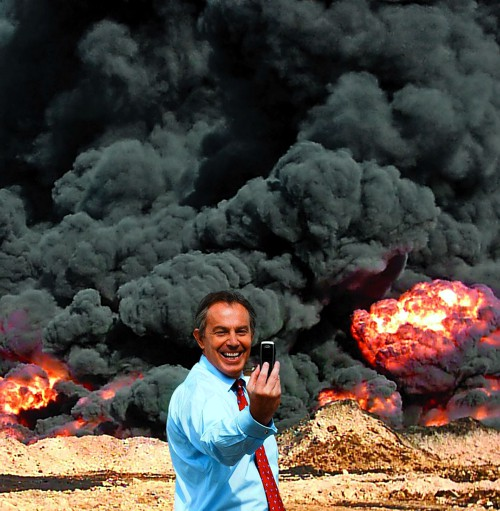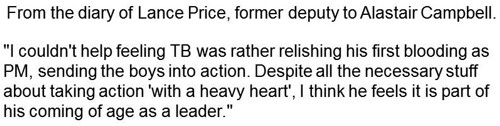Latest Tweets

Every day one sees politicians on TV assuring us that nuclear deterrence works because there no nuclear weapon has been exploded in anger since 1945. They clearly have no understanding of statistics.

With a few plausible assumptions, we can easily calculate that the time until the next bomb explodes could be as little as 20 years.

Be scared, very scared.

The first assumption is that bombs go off at random intervals. Since we have had only one so far (counting Hiroshima and Nagasaki as a single event), this can’t be verified. But given the large number of small influences that control when a bomb explodes (whether in war or by accident), it is the natural assumption to make. The assumption is given some credence by the observation that the intervals between wars are random [download pdf].

If the intervals between bombs are random, that implies that the distribution of the length of the intervals is exponential in shape, The nature of this distribution has already been explained in an earlier post about the random lengths of time for which a patient stays in an intensive care unit. If you haven’t come across an exponential distribution before, please look at that post before moving on.

All that we know is that 70 years have elapsed since the last bomb. so the interval until the next one must be greater than 70 years. The probability that a random interval is longer than 70 years can be found from the cumulative form of the exponential distribution.

If we denote the true mean interval between bombs as $\mu$ then the probability that an intervals is longer than 70 years is

$\text{Prob}\left( \text{interval > 70}\right)=\exp{\left(\frac{-70}{\mu_\mathrm{lo}}\right)}$

We can get a lower 95% confidence limit (call it $\mu_\mathrm{lo}$) for the mean interval between bombs by the argument used in Lecture on Biostatistics, section 7.8 (page 108). If we imagine that $\mu_\mathrm{lo}$ were the true mean, we want it to be such that there is a 2.5% chance that we observe an interval that is greater than 70 years. That is, we want to solve

$\exp{\left(\frac{-70}{\mu_\mathrm{lo}}\right)} = 0.025$

That’s easily solved by taking natural logs of both sides, giving

$\mu_\mathrm{lo} = \frac{-70}{\ln{\left(0.025\right)}}= 19.0\text{ years}$

A similar argument leads to an upper confidence limit, $\mu_\mathrm{hi}$, for the mean interval between bombs, by solving

$\exp{\left(\frac{-70}{\mu_\mathrm{hi}}\right)} = 0.975$
so
$\mu_\mathrm{hi} = \frac{-70}{\ln{\left(0.975\right)}}= 2765\text{ years}$

If the worst case were true, and the mean interval between bombs was 19 years. then the distribution of the time to the next bomb would have an exponential probability density function, $f(t)$,

 $f(t) = \frac{1}{19} \exp{\left(\frac{-70}{19}\right)}$ There would be a 50% chance that the waiting time until the next bomb would be less than the median of this distribution, =19 ln(0.5) = 13.2 years.In summary, the observation that there has been no explosion for 70 years implies that the mean time until the next explosion lies (with 95% confidence) between 19 years and 2765 years. If it were 19 years, there would be a 50% chance that the waiting time to the next bomb could be less than 13.2 years. Thus there is no reason at all to think that nuclear deterrence works well enough to protect the world from incineration.

### Another approach

My statistical colleague, the ace probabilist Alan Hawkes, suggested a slightly different approach to the problem, via likelihood. The likelihood of a particular value of the interval between bombs is defined as the probability of making the observation(s), given a particular value of $\mu$. In this case, there is one observation, that the interval between bombs is more than 70 years. The likelihood, $L\left(\mu\right)$, of any specified value of $\mu$ is thus

$L\left(\mu\right)=\text{Prob}\left( \text{interval > 70 | }\mu\right) = \exp{\left(\frac{-70}{\mu}\right)}$

 If we plot this function (graph on right) shows that it increases with $\mu$ continuously, so the maximum likelihood estimate of $\mu$ is infinity. An infinite wait until the next bomb is perfect deterrence.

But again we need confidence limits for this. Since the upper limit is infinite, the appropriate thing to calculate is a one-sided lower 95% confidence limit. This is found by solving

$\exp{\left(\frac{-70}{\mu_\mathrm{lo}}\right)} = 0.05$

which gives

$\mu_\mathrm{lo} = \frac{-70}{\ln{\left(0.05\right)}}= 23.4\text{ years}$

Summary

The first approach gives 95% confidence limits for the average time until we get incinerated as 19 years to 2765 years. The second approach gives the lower limit as 23.4 years. There is no important difference between the two methods of calculation. This shows that the bland assurances of politicians that “nuclear deterrence works” is not justified.

It is not the purpose of this post to predict when the next bomb will explode, but rather to point out that the available information tells us very little about that question. This seems important to me because it contradicts directly the frequent assurances that deterrence works.

The only consolation is that, since I’m now 79, it’s unlikely that I’ll live long enough to see the conflagration.

Anyone younger than me would be advised to get off their backsides and do something about it, before you are destroyed by innumerate politicians.

Postscript

While talking about politicians and war it seems relevant to reproduce Peter Kennard’s powerful image of the Iraq war.and with that, to quote the comment made by Tony Blair’s aide, Lance PriceIt’s a bit like my feeling about priests doing the twelve stations of the cross. Politicians and priests masturbating at the expense of kids getting slaughtered (at a safe distance, of course).